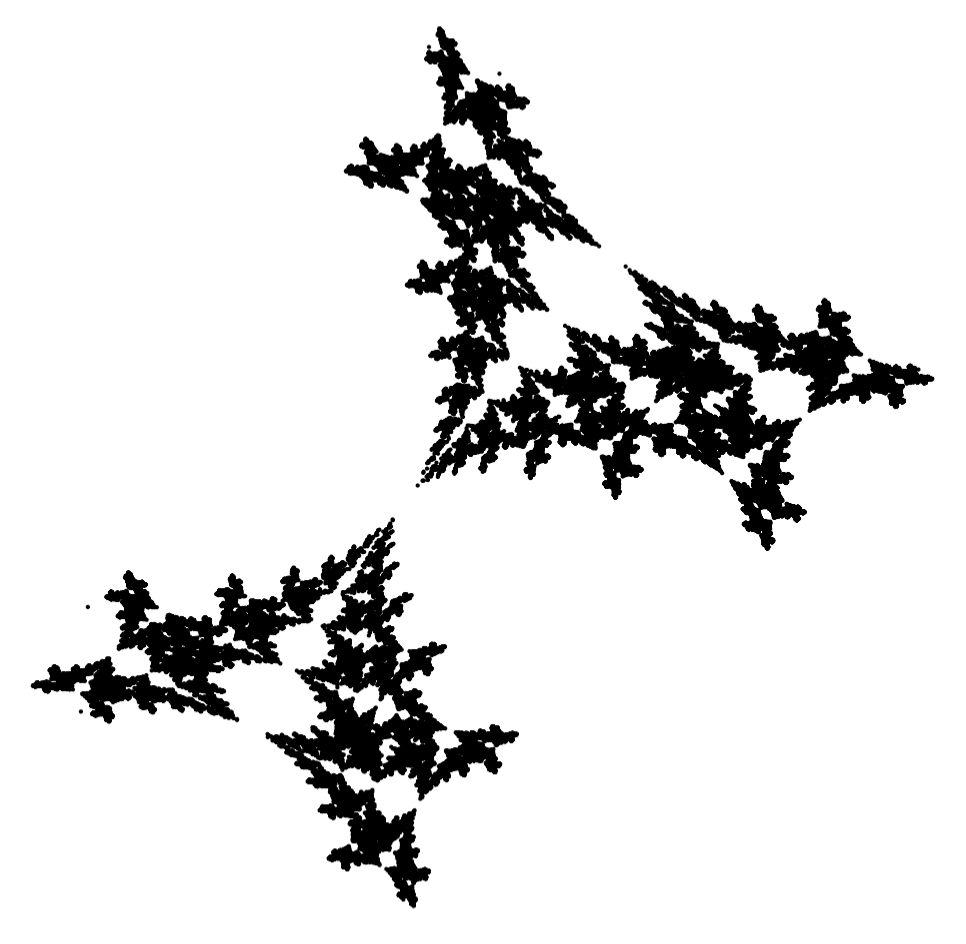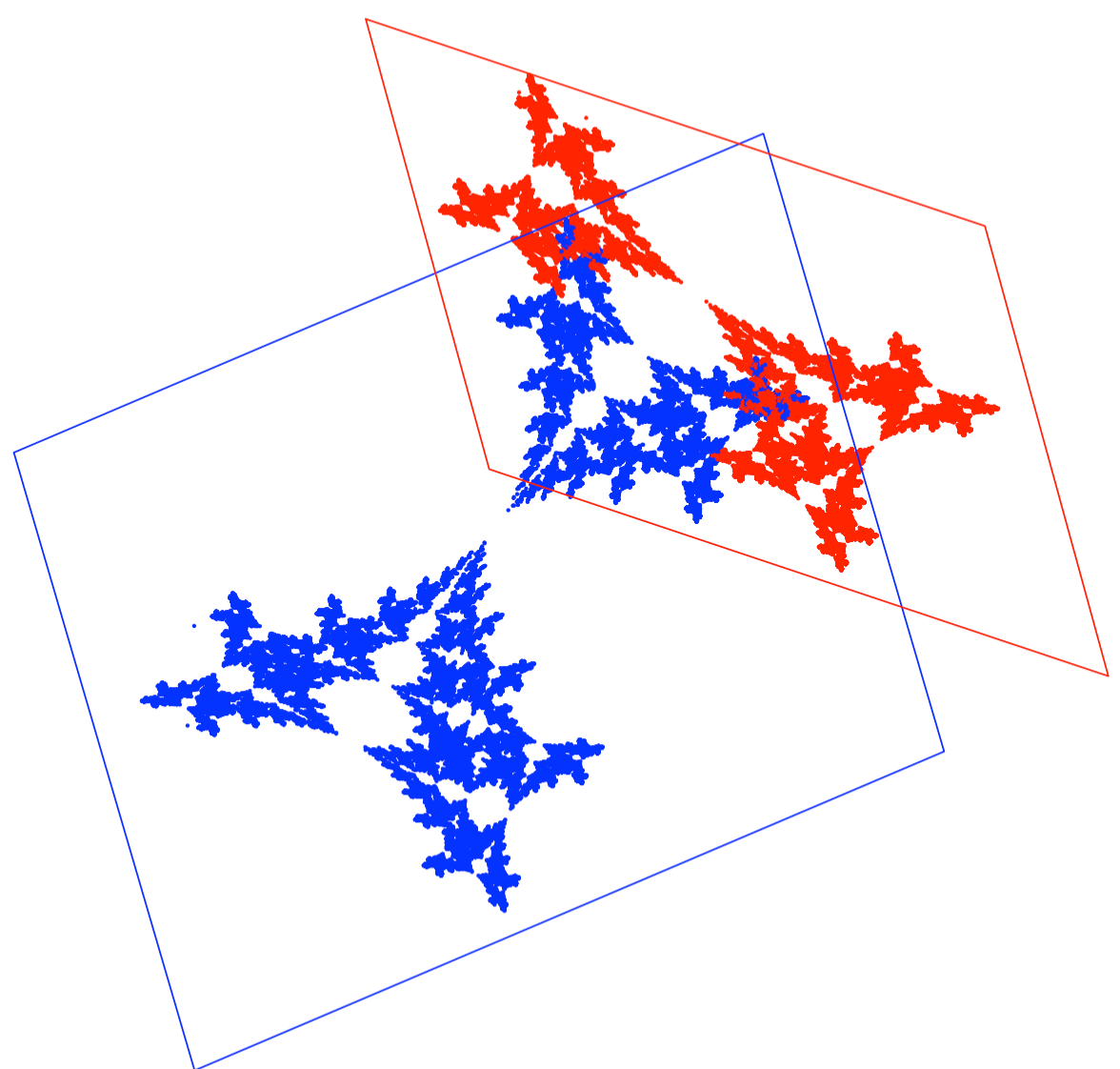## 4.8 Sky is the limitThe number of fractals, even those built from two transformations, is literally infinite.

It is worth experimenting with them!$f_1(x, y) = \begin{bmatrix} 0.202 & -0.805 & -0.373 \\ -0.689 & -0.342 & -0.653 \end{bmatrix} [x \ y \ 1]^T$ $f_2(x, y) = \begin{bmatrix} 0.138 & 0.665 & 0.66 \\ -0.502 & -0.222 & -0.277 \end{bmatrix} [x \ y \ 1]^T$